International
Tables for
Crystallography
Volume B
Reciprocal space
Edited by U. Shmueli

International Tables for Crystallography (2006). Vol. B. ch. 2.1, p. 196   | 1 | 2 |

## Section 2.1.5.5. Relation to distributions of I

U. Shmuelia* and A. J. C. Wilsonb

aSchool of Chemistry, Tel Aviv University, Tel Aviv 69 978, Israel, and bSt John's College, Cambridge, England
Correspondence e-mail:  ushmueli@post.tau.ac.il

#### 2.1.5.5. Relation to distributions of I

| top | pdf |

When only the intrinsic probability distributions are being considered, it does not greatly matter whether the variable chosen is the intensity of reflection (I), or its positive square root, the modulus of the structure factor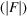, since both are necessarily real and non-negative. In an obvious notation, the relation between the intensity distribution and the structure-factor distribution is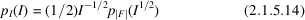or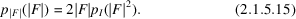Statistical fluctuations in counting rates, however, introduce a small but finite probability of negative observed intensities (Wilson, 1978a, 1980a) and thus of imaginary structure factors. This practical complication is treated in IT C (2004, Parts 7and 8).

Both the ideal centric and acentric distributions are simple members of the family of gamma distributions, defined by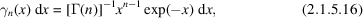where n is a parameter, not necessarily integral, and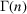is the gamma function. Thus the ideal acentric intensity distribution is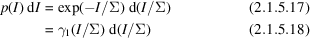and the ideal centric intensity distribution is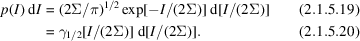The properties of gamma distributions and of the related beta distributions, summarized in Table 2.1.5.1, are used in Section 2.1.6to derive the probability density functions of sums and of ratios of intensities drawn from one of the ideal distributions.

 Table 2.1.5.1| top | pdf | Some properties of gamma and beta distributions
 If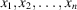are independent gamma-distributed variables with parameters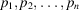, their sum is a gamma-distributed variable with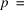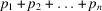. If x and y are independent gamma-distributed variables with parameters p and q, then the ratio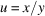has the distribution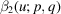. With the same notation, the ratio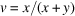has the distribution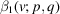. Differences and products of gamma-distributed variables do not lead to simple results. For proofs, details and references see Kendall & Stuart (1977).
Name of the distribution, its functional form, mean and variance
Gamma distribution with parameter p: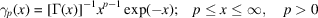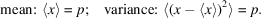Beta distribution of first kind with parameters p and q: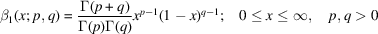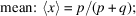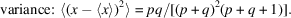Beta distribution of second kind with parameters p and q: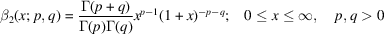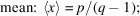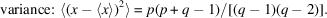### ReferencesInternational Tables for Crystallography (2004). Vol. C. Mathematical, physical and chemical tables, edited by E. Prince. Dordrecht: Kluwer Academic Publishers.Google ScholarKendall, M. & Stuart, A. (1977). The advanced theory of statistics, Vol. 1, 4th ed. London: Griffin.Google ScholarWilson, A. J. C. (1978a). On the probability of measuring the intensity of a reflection as negative. Acta Cryst. A34, 474–475.Google ScholarWilson, A. J. C. (1980a). Relationship between observed' and true' intensity: effects of various counting modes. Acta Cryst. A36, 929–936.Google Scholar